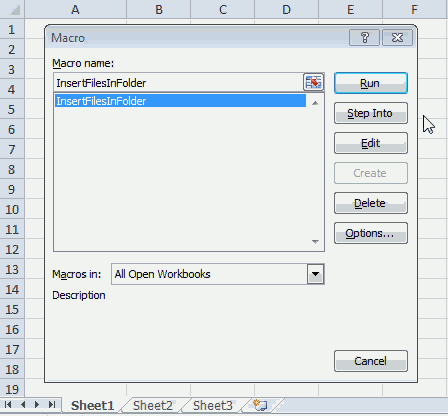Author: Oscar Cronquist Article last updated on September 25, 2017The macro creates a new sheet. Inserts all filenames in current folder as hyperlinks except the current workbook.

### VBA Code

```Sub InsertFilesInFolder()
Dim sPath As String, Value As String
Dim WS As Worksheet
sPath = ActiveWorkbook.Path & "\"
Value = Dir(sPath, &H1F)
WS.Range("A1") = "Filename"
Set StartCell = WS.Range("A2")
Do Until Value = ""
If Value = "." Or Value = ".." Then
Else
If GetAttr(sPath & Value) = 16 Then
Else
If Value <> ActiveWorkbook.Name And Value <> "~\$" & ActiveWorkbook.Name Then